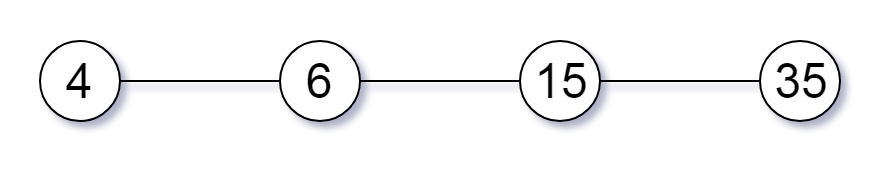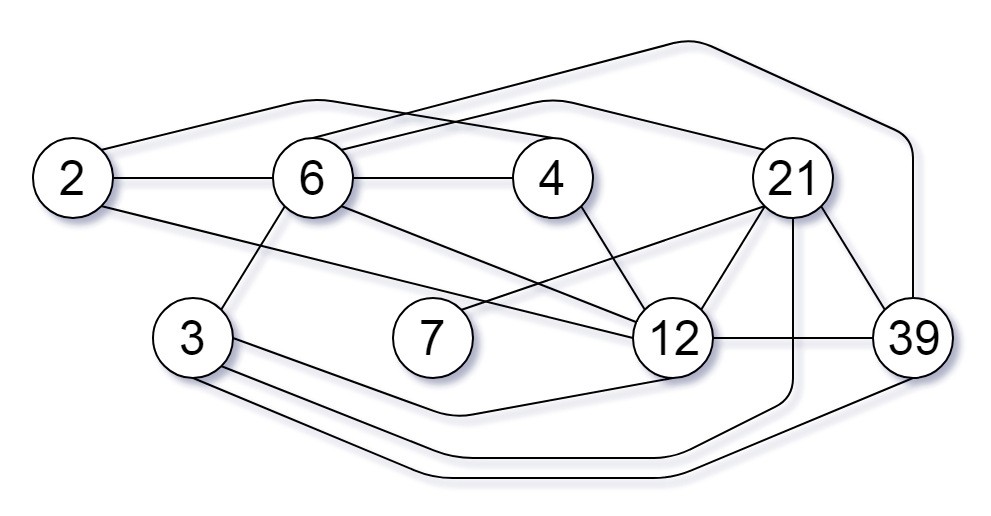# 952.largest component size by common factor(按公因数计算最大组件大小)## Description

Given a non-empty array of unique positive integers A, consider the following graph:

• There are A.length nodes, labelled A to A[A.length - 1];
• There is an edge between A[i] and A[j] if and only if A[i] and A[j] share a common factor greater than 1.

Return the size of the largest connected component in the graph.

• 有 A.length 个节点，按从 A 到 A[A.length - 1] 标记；
• 只有当 A[i] 和 A[j] 共用一个大于 1 的公因数时，A[i] 和 A[j] 之间才有一条边。

### Example 1:

``````Input: [4,6,15,35]
Output: 4
``````### Example 2:

``````Input: [20,50,9,63]
Output: 2
``````### Example 3:

``````Input: [2,3,6,7,4,12,21,39]
Output: 8
``````### Note:

• 1 <= A.length <= 20000
• 1 <= A[i] <= 100000

1. updating

## 参考代码

``````updating
``````